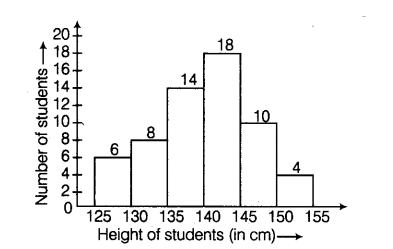# Look at the histogram below and

Question:

Look at the histogram below and answer the questions that follow.(a) How many students have height more than or equal to 135 cm, but less than 150 cm ?

(b) Which class interval has the least number of students?

(c) What is class size?

(d) How many students have height less than 140 cm?

Solution:

(a) Number of students who have height more than or equal to 135 cm, but less than 150 cm = 14+ 18+ 10 = 42

(b) The class interval 150-155 has the least number of students, i.e. 4.

(c) We-know, class size = Upper class limit – Lower class limit Consider any class, say (125-130), then class size = 130-125 = 5 Hence, the class

size is 5.

(d) Number of students who have height less than 140 cm = 6 + 8 + 14 = 28